TEST Statement

<label:> TEST equation <,, equation> </ options> ;

The TEST statement tests linear hypotheses about the regression coefficients. PROC PHREG performs a Wald test for the joint hypothesis specified in a single TEST statement. Each equation specifies a linear hypothesis; multiple equations (rows of the joint hypothesis) are separated by commas. The label, which must be a valid SAS name, is used to identify the resulting output and should always be included. You can submit multiple TEST statements.

The form of an equation is as follows:

term <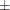term …> <= <term <term …>>>

where term is a variable or a constant or a constant times a variable. The variable is any explanatory variable in the MODEL statement. When no equal sign appears, the expression is set to 0. The following program illustrates possible uses of the TEST statement:

proc phreg;
model time= A1 A2 A3 A4;
Test1: test A1, A2;
Test2: test A1=0,A2=0;
Test3: test A1=A2=A3;
Test4: test A1=A2,A2=A3;
run;

Note that the first and second TEST statements are equivalent, as are the third and fourth TEST statements.

The following options can be specified in the TEST statement after a slash (/):

AVERAGE

enables you to assess the average effect of the variables in the given TEST statement. An overall estimate of the treatment effect is computed as a weighted average of the treatment coefficients as illustrated in the following statement:

TREATMENT: test trt1, trt2, trt3, trt4 / average;

Let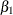,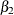,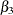, and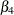be corresponding parameters for trt1, trt2, trt3, and trt4, respectively. Let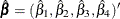be the estimated coefficient vector and let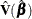be the corresponding variance estimate. Assuming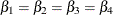, let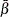be the average treatment effect. The effect is estimated by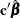, where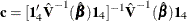and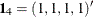. A test of the null hypothesis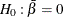is also included, which is more sensitive than the multivariate test for testing the null hypothesis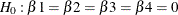.

E

specifies that the linear coefficients and constants be printed. When the AVERAGE option is specified along with the E option, the optimal weights of the average effect are also printed in the same tables as the coefficients.

PRINT

displays intermediate calculations. This includes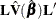bordered by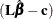, and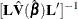bordered by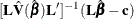, whereis a matrix of linear coefficients andis a vector of constants.

See the section Using the TEST Statement to Test Linear Hypotheses for details.